# Test Prep Plan - Take a practice test

Take this practice test to check your existing knowledge of the course material. We'll review your answers and create a Test Prep Plan for you based on your results.
How Test Prep Plans work
1
2Based on your results, we'll create a customized Test Prep Plan just for you!
3Study smarter
Study more effectively: skip concepts you already know and focus on what you still need to learn.

# NMTA Middle Grades Math: Circular Arcs & Measurement Chapter Exam

Exam Instructions:

Choose your answers to the questions and click 'Next' to see the next set of questions. You can skip questions if you would like and come back to them later with the yellow "Go To First Skipped Question" button. When you have completed the practice exam, a green submit button will appear. Click it to see your results. Good luck!

### Page 1

#### Question 3 3. If the measure of central angle AOB is 62 degrees, what is the measure of inscribed angle ACB?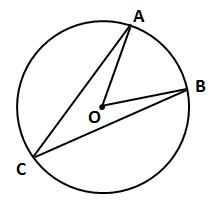### Page 2

#### Question 6 6. In the picture below, if arc BD is 168 degrees and arc BC is 70 degrees, what is the measure of angle BAD?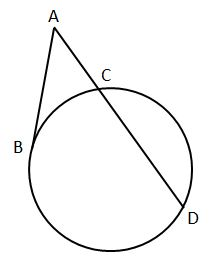#### Question 7 7. In the picture below, if AE is 10, AB is 14 and ED is 16, what is the length of CE?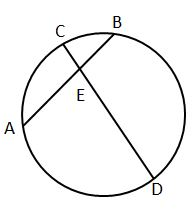#### Question 8 8. If arc AC is 106 degrees and arc BC is 82 degrees, what is the measure of angle ACB?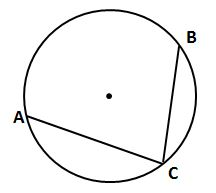#### Question 10 10. What is the area of the shaded area, rounded to the nearest whole number?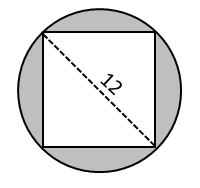### Page 3

#### Question 12 12. If AB and CB are tangent segments, what is the length of CB?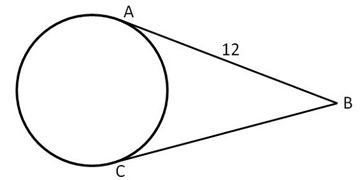### Page 4

#### Question 18 18. In the picture below, if arc CE is 172 degrees and arc BD is 80 degrees, what is the measure of angle CAE?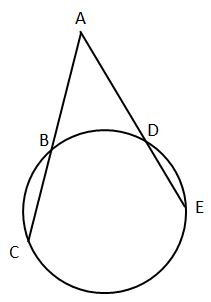#### Question 19 19. In the picture below, if AC is 14 and AB is 10, what is the length of AD?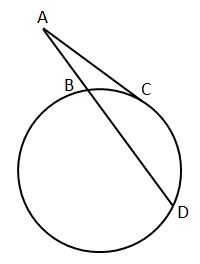#### Question 20 20. In the picture below, if AB is 7, BC is 19 and AD is 8, what is the length of DE?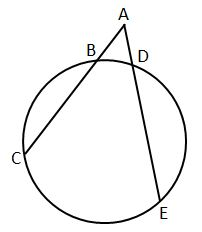### Page 5

#### Question 23 23. In the picture below, if arc AB is 82 degrees, what is the measure of angle BAC?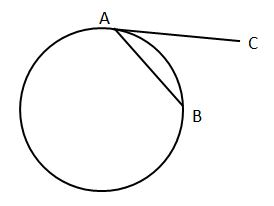#### Question 24 24. In the picture below, if AE is 8, CE is 12 and ED is 5, what is the length of EB?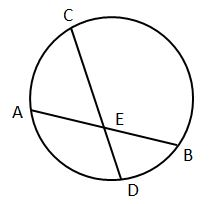#### Question 25 25. Which line is a tangent to the pictured circle?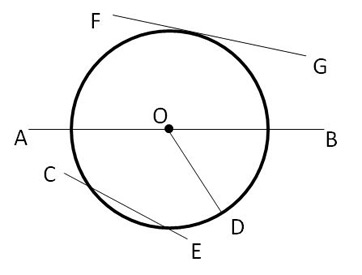### Page 6

#### Question 26 26. In the picture below, if AB is 12, AC is 24 and AD is 10, what is the length of AE?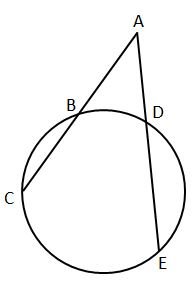#### Question 28 28. If arc AC is 122 degrees and arc BC is 192 degrees, what is the measure of angle ACB?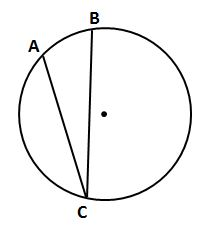#### Question 30 30. In the picture below, if major arc BC is 258 degrees and minor arc BC is 102 degrees, what is the measure of angle BAC?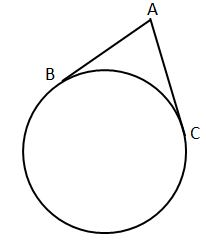#### NMTA Middle Grades Math: Circular Arcs & Measurement Chapter Exam Instructions

Choose your answers to the questions and click 'Next' to see the next set of questions. You can skip questions if you would like and come back to them later with the yellow "Go To First Skipped Question" button. When you have completed the practice exam, a green submit button will appear. Click it to see your results. Good luck!

Support